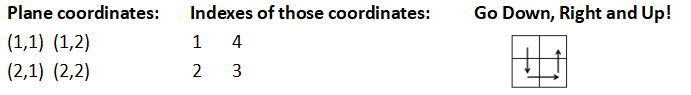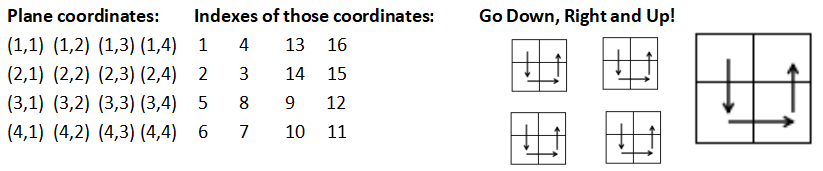Problem E: Down, Right and Up!

## Problem E: Down, Right and Up!

Time Limit: 4 Sec  Memory Limit: 128 MB
Submit: 769  Solved: 144
[Submit][Status][Web Board]

## Description

How to count an infinity set? For example, all the points in a 2-D space which have positive integer coordinates. lanran finds a way to solve that, ‘Down, Right and Up!’ Let’s see how we count first several points by the above way.How do we count more?Hopefully, you have understood this procedure well. Similarly, we can expand the ‘matrix’ to infinitely large, that is, we can count all the points which have positive integer coordinates in a 2-D space.

Given a coordinate, you need to return its index and given an index, you need to return its coordinate.

## Input

The first line of input is the number of test cases T (1<=T<=100,000)
For each test case, there could only be a coordinate,(x,y) or an index a.(1<=x,y<=109 , 1<=a<=1018) You may need 'long long' in C++, or 'long' in Java to store the value of a.

## Output

The coordinate or index that matches each query.

## Sample Input

2
1
(1,2)

## Sample Output

(1,1)
4

[Submit][Status]